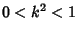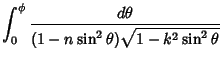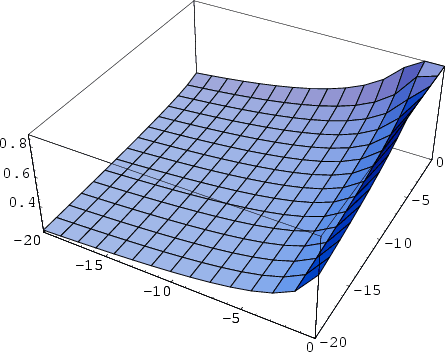## Elliptic Integral of the Third Kind

Let. The incomplete elliptic integral of the third kind is then defined as(1)(2)

whereis a constant known as the Characteristic.The complete elliptic integral of the second kind(3)

is illustrated above.

See also Elliptic Integral of the First Kind, Elliptic Integral of the Second Kind, Elliptic Integral Singular Value

References

Abramowitz, M. and Stegun, C. A. (Eds.). Elliptic Integrals'' and Elliptic Integrals of the Third Kind.'' Ch. 17 and §17.7 in Handbook of Mathematical Functions with Formulas, Graphs, and Mathematical Tables, 9th printing. New York: Dover, pp. 587-607, 1972.